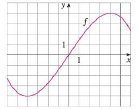Chapter 1, Problem 1RE### Single Variable Calculus: Early Tr...

8th Edition
James Stewart
ISBN: 9781305270343

#### Solutions

Chapter
Section### Single Variable Calculus: Early Tr...

8th Edition
James Stewart
ISBN: 9781305270343
Textbook Problem

# Let f be the function whose graph is given.(a) Estimate the value of f(2).(b) Estimate the values of x such that f(x) = 3.(c) State the domain of f(d) State the range of f.(e) On what interval is f increasing?(f) Is f one-to-one? Explain.(g) Is f even, odd, or neither even nor odd? Explain.

(a)

To determine

To estimate: The value of f(2).

Explanation

Let y=f(x) be the function.

From the given graph, it is noticed that when the curve approaches x

(b)

To determine

To estimate: The value of x when f(x)=3.

(c)

To determine

To state: The domain of f.

(d)

To determine

To state: The range of f.

(e)

To determine

To find: The interval on which f is increasing.

(f)

To determine

To check: Whether the function is one-to-one and explain the reason.

(g)

To determine

To check: Whether the function is even, odd or neither even nor odd.

### Still sussing out bartleby?

Check out a sample textbook solution.

See a sample solution

#### The Solution to Your Study Problems

Bartleby provides explanations to thousands of textbook problems written by our experts, many with advanced degrees!

Get Started

#### Expand each expression in Exercises 122. (4y2)y

Finite Mathematics and Applied Calculus (MindTap Course List)

#### In Exercises 16, simplify the expression. 6. (1+x2)2(2)2x(2)(1+x2)(2x)(1+x2)4

Applied Calculus for the Managerial, Life, and Social Sciences: A Brief Approach

#### In problems 45-48, determine a value for that makes each statement true. 48.

Mathematical Applications for the Management, Life, and Social Sciences

#### Calculate y'. 22. y = sec(1 + x2)

Single Variable Calculus: Early Transcendentals